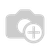100 Product(s) Found

## Ceramics & Pottery

Ceramics and pottery have their roots in Gandhara civilization, even the ruins of Gandhara have yielded toys made with clay and this tradition has stayed alive in the villages of southern Punjab and Sindh where you would find artisans creating ceramics and then painting them over with Niqashi or Kashi Kari.
1,300.00 Rs.
1,300.00 Rs.
1,300.00 Rs.
900.00 Rs.
900.00 Rs.
900.00 Rs.
900.00 Rs.
900.00 Rs.
900.00 Rs.
900.00 Rs.
1,000.00 Rs.
550.00 Rs.
800.00 Rs.
2,150.00 Rs.
700.00 Rs.
700.00 Rs.
3,500.00 Rs.
2,000.00 Rs.
500.00 Rs.
1,520.00 Rs.
500.00 Rs.
500.00 Rs.
400.00 Rs.
500.00 Rs.
500.00 Rs.
500.00 Rs.
500.00 Rs.
500.00 Rs.
500.00 Rs.
500.00 Rs.
500.00 Rs.
500.00 Rs.
500.00 Rs.
500.00 Rs.
400.00 Rs.
400.00 Rs.
400.00 Rs.
400.00 Rs.
6,500.00 Rs.
6,500.00 Rs.
4,300.00 Rs.
6,500.00 Rs.
6,500.00 Rs.
6,500.00 Rs.
4,300.00 Rs.
4,300.00 Rs.
6,500.00 Rs.
5,000.00 Rs.
1,800.00 Rs.
700.00 Rs.
1,200.00 Rs.
1,000.00 Rs.
1,500.00 Rs.
750.00 Rs.
3,500.00 Rs.
1,199.00 Rs.
1,250.00 Rs.
1,600.00 Rs.
1,100.00 Rs.
790.00 Rs.
1,500.00 Rs.
890.00 Rs.
890.00 Rs.
900.00 Rs.
900.00 Rs.
1,500.00 Rs.
1,750.00 Rs.
1,500.00 Rs.
900.00 Rs.
1,700.00 Rs.
1,980.00 Rs.
880.00 Rs.
850.00 Rs.
1,450.00 Rs.
850.00 Rs.
1,000.00 Rs.
1,100.00 Rs.
650.00 Rs.
2,700.00 Rs.
7,200.00 Rs.
1,300.00 Rs.
1,300.00 Rs.
1,300.00 Rs.
750.00 Rs.
1,250.00 Rs.
1,000.00 Rs.
1,000.00 Rs.
1,700.00 Rs.
1,200.00 Rs.
1,200.00 Rs.
1,200.00 Rs.
1,200.00 Rs.
1,200.00 Rs.
1,200.00 Rs.
1,200.00 Rs.
1,200.00 Rs.
1,200.00 Rs.
1,390.00 Rs.
1,390.00 Rs.
###### The Volkswagon Foxy Planter
890.00 Rs.To install this Web App in your iPhone/iPad pressand then Add to Home Screen.